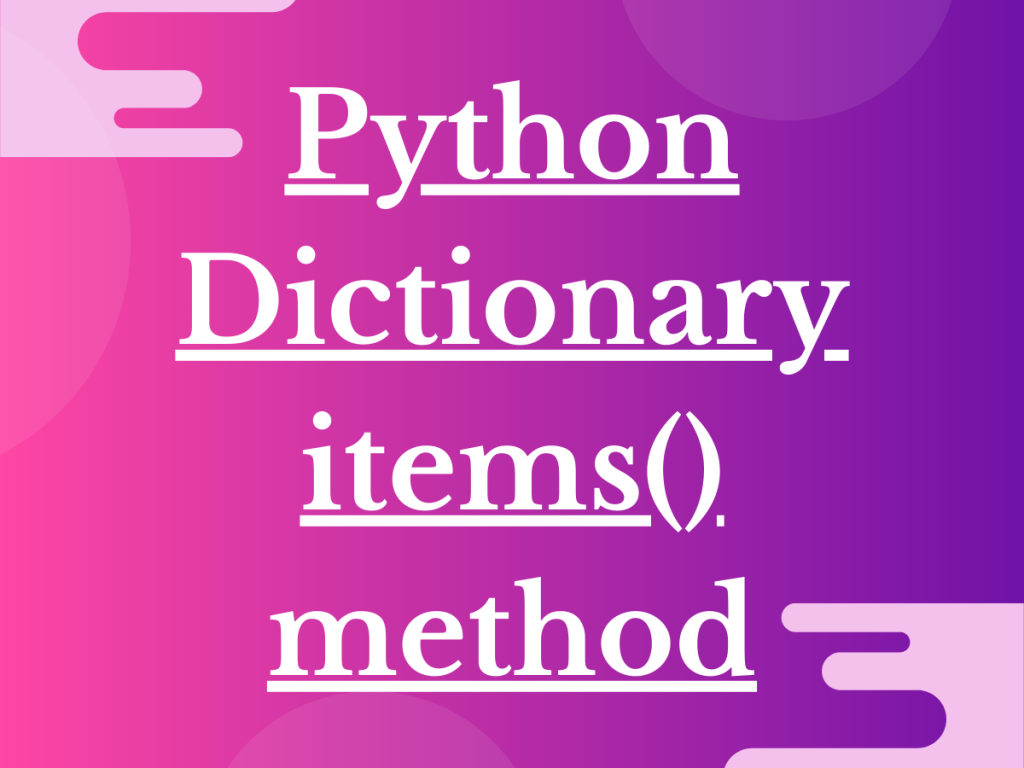# 5 Examples of Python Dict items() methodHey, folks! In this article, we will be focusing on Python Dict items() method to extract and represent the dictionary values.

## How does Python Dict items() method work?

Python Dictionary is a data structure that stores data elements in a key-value pair format. Further, every unique key is associated with unique/redundant values.

In order to iterate and display the dictionary elements, the Python dict items() method serves the purpose.

The `dict.items() method` displays the data elements occupied by a dictionary as a list of key-value pairs.

Syntax:

```dict.items()
```

The dict.items() method does not accept any parameter and returns a list containing the key-value as tuple pairs of the dictionary.

### Returning a List of (Key, Value) pairs using Python Dict items()

In this example, we have created a dictionary and used the dict.items() method to return the list of all the key-value pairs present in the input dictionary.

```inp_dict = { 'a':3,'ab':2,'abc':1,'abcd':0 }
print("Dictionary: ", inp_dict.items())

```

Output:

```Dictionary:  dict_items([('a', 3), ('ab', 2), ('abc', 1), ('abcd', 0)])
```

### Using Python Dict items to Return String Items

In the below example, the input dictionary contains key-value pairs in the form of string values.

Thus, it can be said that the `dict.items() method` does not get affected by the type of key or value and returns a list of all the elements present in the dict as tuple pairs.

```lang_dict = {
'A':'Python',
'B':'C++',
'C':'Kotlin'}
print("Dictionary: ", lang_dict.items())

```

Output:

```Dictionary:  dict_items([('A', 'Python'), ('B', 'C++'), ('C', 'Kotlin')])
```

### Returning Empty Dictionary using Dict items()

The dict.items() method does not throw any kind of error or exception for an empty dictionary. Thus, if a dictionary is empty, the dict.items() method returns an empty list.

```empty_dict = {}
print("Dictionary: ", empty_dict.items())

```

Output:

```Dictionary:  dict_items([])
```

### Printing Out Dictionary Items After Updating Values

In the below example, we have created an input dictionary and have displayed the dict values using items() function.

Further, we have updated the dictionary key-value pair and displayed the dict using items() method again. Thus, it can be said that the dict.items() method goes well along with the updation of dictionary and displays the updated values in the list format.

If the dict gets updated, the changes are automatically reflected in the list displayed by using the dict.input() method.

```lang_dict = {
'A':'Python',
'B':'C++',
'C':'Kotlin'}
print("Dictionary before updation: ", lang_dict.items())
lang_dict['B']='Ruby'
print("Dictionary after updation: ", lang_dict.items())

```

Output:

```Dictionary before updation:  dict_items([('A', 'Python'), ('B', 'C++'), ('C', 'Kotlin')])
Dictionary after updation:  dict_items([('A', 'Python'), ('B', 'Ruby'), ('C', 'Kotlin')])
```

### Printing Out Dictionary Elements Without Dict items() method

We can display the elements of the dictionary directly in the key-value form by calling the input dictionary object as shown below:

```input_dict={}
print(input_dict)
```

The above line of code would return an empty parenthesis ‘{}’ as a result.

In the below example, we have used the dictionary object and the dict.items() method to display the dict key-value pairs.

```lang_dict = {
'A':'Python',
'B':'C++',
'C':'Kotlin'}
print("Dictionary: ", lang_dict)
print("Dictionary using items() method:", lang_dict.items())

```

Output:

```Dictionary:  {'A': 'Python', 'B': 'C++', 'C': 'Kotlin'}
Dictionary using items() method: dict_items([('A', 'Python'), ('B', 'C++'), ('C', 'Kotlin')])
```

## Summary

• The Python dict.items() method is used to display the data elements of the dict in the form of a list of tuple pairs.
• If a dict is empty, the dict.items() method returns an empty list.
• When we update a dictionary, the changes are well incorporated in the output list by the dict.items() method.

## Conclusion

Thus, in this article, we have understood the working of Python Dictionary items() method against various examples.